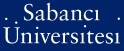# A Kronecker's limit formula for real quadratic number fieldsThe system is temporarily closed to updates for reporting purpose.

Kalaycı, Serhan (2005) A Kronecker's limit formula for real quadratic number fields. [Thesis]Preview
PDF - Requires a PDF viewer such as GSview, Xpdf or Adobe Acrobat Reader
568Kb

## Abstract

Let K be a quadratic number field, the Dedekind zeta-function of K, (k(s) can be written as a sum of partial zeta functions, Ł(s, ̂4) where A runs over the ideal class group of K and s a complex number. Then Ł(s, ̂4) has an analytic continuation as a meromorphic function of s with a simple pole at s = 1. Dirichlet proved that the residue of ((s,A) is independent of the ideal class A chosen. For the constant in the Laurent expansion of partial zeta function around s = 1 we will examine Kronecker's and Zagier's results. Kronecker found the constant for the imaginary quadratic case. Working with imaginary quadratic fields is much easier because of the finiteness of unit group of the field. For real quadratic fields there are infinitely many units and Zagier computed the constant for this case. Also we will include continued fractions as Zagier used for the proof of the limit formula of zeta-function for real quadratic number fields.

Item Type: Thesis Quadratic number fields. -- Zeta functions. -- Continued fractions. -- Ideal class Q Science > QA Mathematics 8245 IC-Cataloging 16 Apr 2008 15:54 25 Mar 2019 16:52

Repository Staff Only: item control page